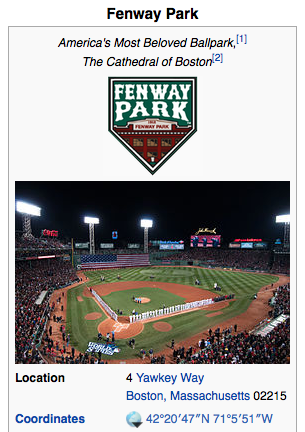## Sunday, December 27, 2015

### 11 74 111 139 216 666 | First Ever Baseball Game at Fenway Park, April 20, 1912, Against the New York Highlanders

Fenway, a name that needs no introduction at this day in age.

Fenway = 6+5+14+23+1+25 = 74
Masonic = 13+1+19+15+14+9+3 = 74

To make no mistake about it, the name Fenway is definitely a tribute to the Masons.  Look at the address of the ballpark, Yawkey Way.

Yawkey = 25+1+23+11+5+25 = 90
Way = 23+1+25 = 49
Yawkey Way = 139
Freemasonry = 6+18+5+5+13+1+19+15+14+18+25 = 139

Notice that the first ever game played at Fenway was between the New York Highlanders (now named the Yankees), on April 20, 1912.  1912 was a leap year, so April 20 was the 111th day of the year, much like the gematria of New York.

New York =14+5+23+25+15+18+11 = 111 (666 in English Gematria)
Highlanders = 8+9+7+8+12+1+14+4+5+18+19 = 105
New York Highlanders = 216 (6x6x6 = 216)Freemason = 42

You have to love that the first game went to the 11th inning, against the team from the 11th state, New York, on the date with '111' connections.  Baseball, it has always been by the numbers, and that is why I was able to call the Mets in the 111th World Series in Spring Training.